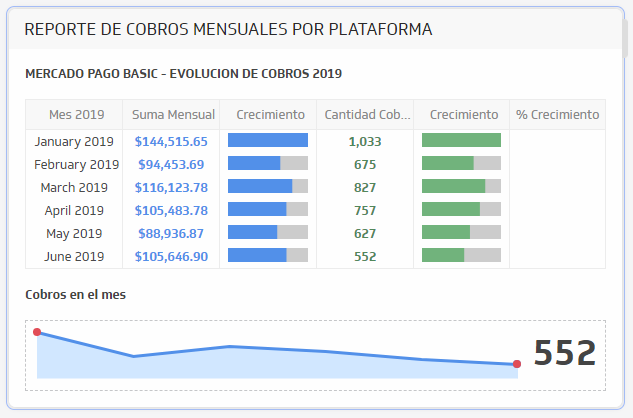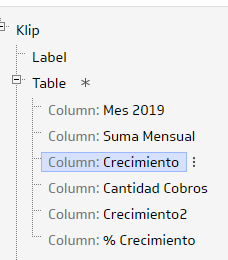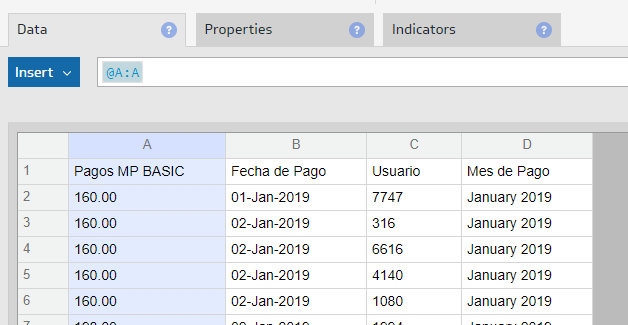Hi.

I have this Table, with this columns:

• Grouped months
• Sum Prices by months
• Mini Chart Horizontal Sum Prices
• Count of payments by monts
• Mini Chart Horizontal Count paymentsWhat I need to do, in the sixth column that is empty, is to indicate the percentage of monthly growth.

Example:

January: 1033 payments
February: 675 payments

Expected result, for the February monthly growth (%) = (675-1033) / 1033  =  -34%

How can I do this, with a formula?

Best Regards

#### 1 comment

•Janice Janczyn

Hi Teatrix,

If you are using Applied Actions to group and aggregate the table columns, you need to use Results References to calculate a ratio or percentage so that the ratio is calculated based on the aggregated values. To calculate a delta change, you would use this formula

(current value - previous value) / previous value = current value / previous value - 1

Following your example, you would use Results Reference to refer to the Cantidad Cobros column.